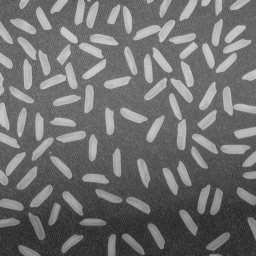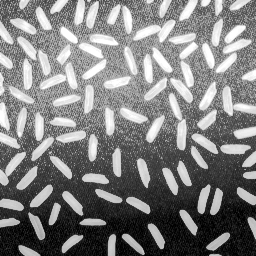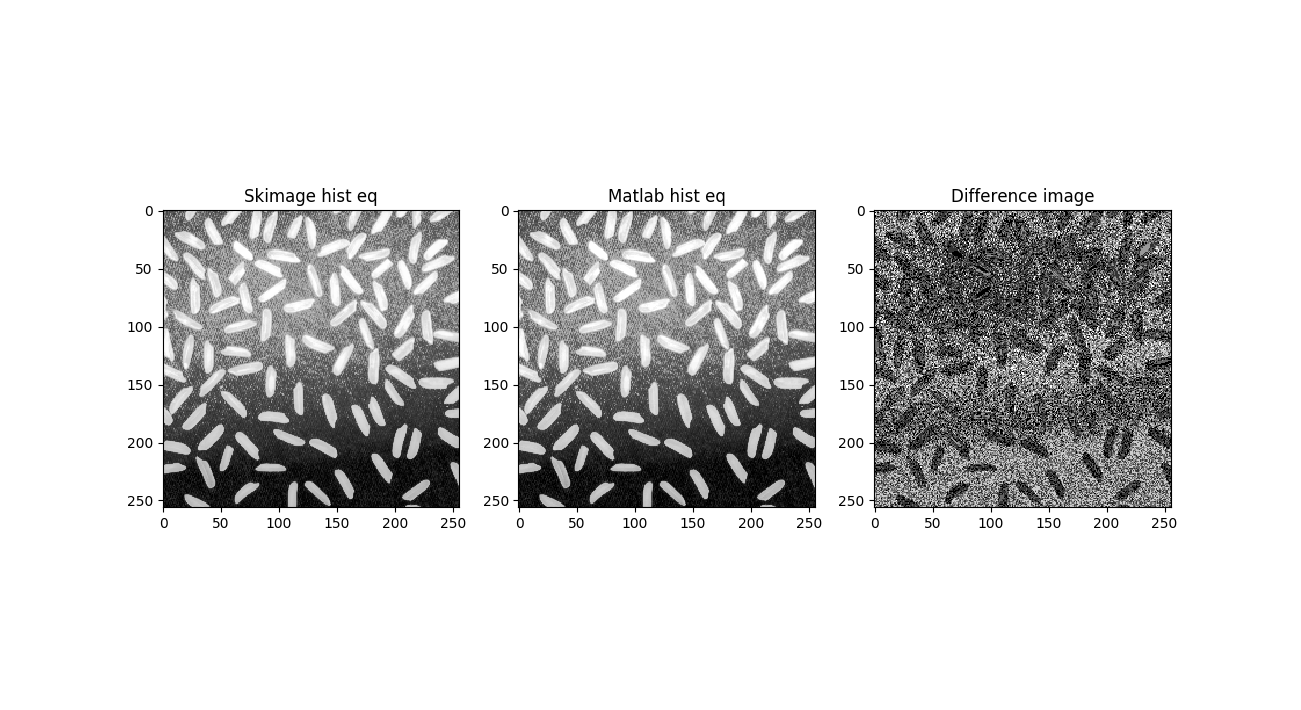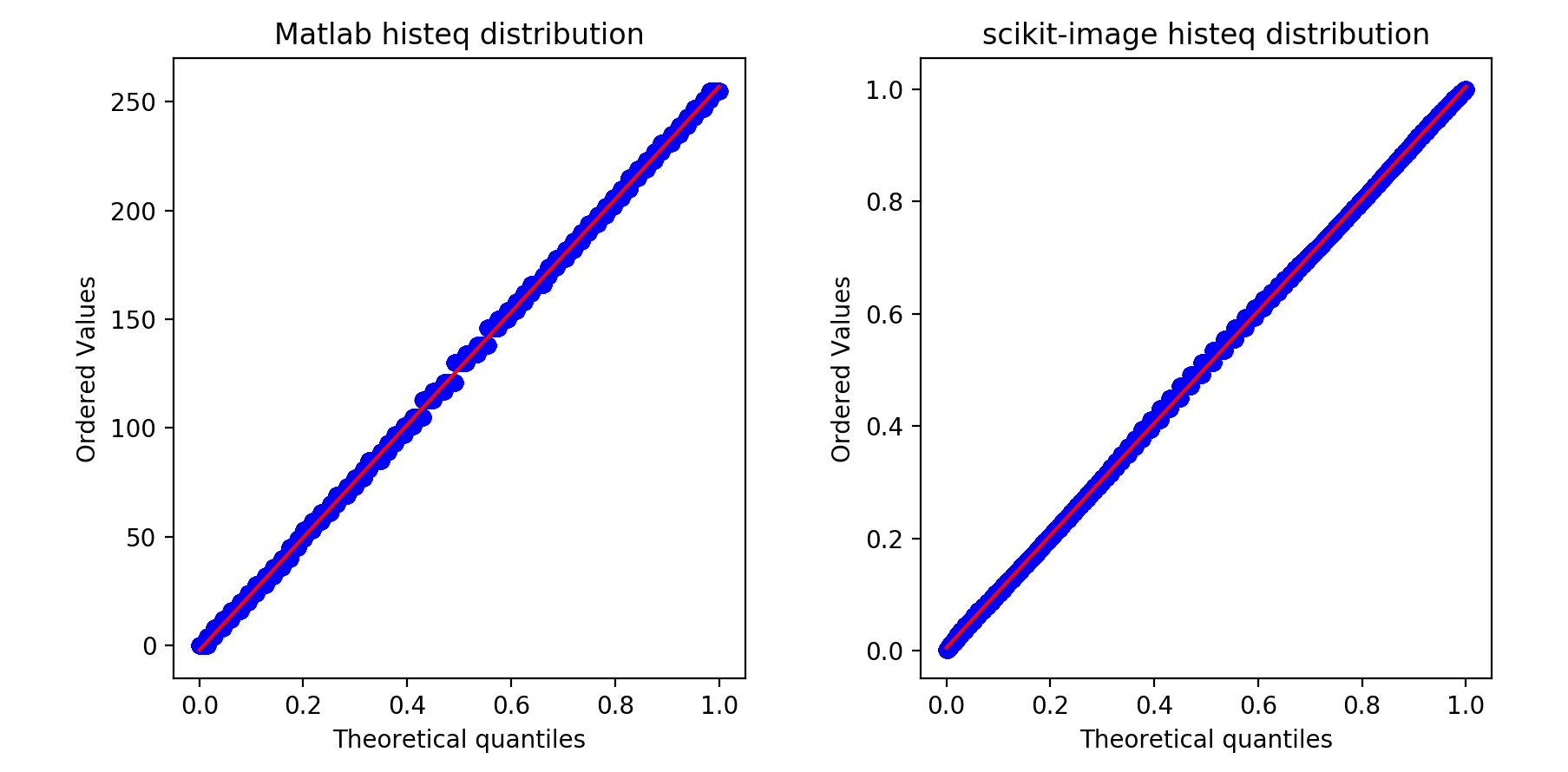{{ message }}

# exposure.equalize_hist doesn't output the same type as the input #2677

Closed
opened this issue Jun 10, 2017 · 6 comments
Closed

# exposure.equalize_hist doesn't output the same type as the input#2677

opened this issue Jun 10, 2017 · 6 comments
Labels

###TheShadow29 commented Jun 10, 2017 • edited

 I am not completely sure if it should be a feature request or a bug. Currently exposure.equalize_hist given an image of input of type uint8 outputs an image of type float64. The proposal is to give the output in uint8 format itself. Current implementation: ``````img1 = io.imread(path_to_file) img11 = skimage.exposure.equalize_hist(img1) print('Original Image is of dtype ', img1.dtype) print('Histogram Equalized Image is of dtype', img11.dtype) `````` Output: ``````('Original Image is of dtype ', dtype('uint8')) ('Histogram Equalized Image is of dtype', dtype('float64')) `````` #1192 mentions this, but the issue is not really addressed. To be honest I am not even sure why the output image is of type 'float64'. At no point of time should image of type uint8 should be changed to float64, i.e. there should be no case where we should be working with floats, we should be in integer domain itself. Here is a nice text describing histogram equalization https://www.math.uci.edu/icamp/courses/math77c/demos/hist_eq.pdf Histogram equalization would simply be a transformation curve, mapping one intensity to another corresponding intensity (the formulae given in the mentioned link) and it should be all in the integer domain when the input is of type uint8. Also as far as I know, MATLAB also does the same thing, that is given an input of uint8 returns output in uint8 itself. Link for the same https://www.mathworks.com/help/images/ref/histeq.html In short, the current implementation of the equalize_hist is doing something similar but will give a different result from what a normal histogram equalization would return at least for an uint8 image. Also I think it is more sensible to return image of the same dtype. Here is a matlab vs skimage comparison. Code for matlab ``````img = imread('rice.png'); im1 = histeq(img); imwrite(img, 'rice.png'); imwrite(im1, 'rice_eq.png'); `````` Now for the skimage code. ``````%matplotlib import skimage from skimage import io import matplotlib.pyplot as plt io.use_plugin('matplotlib') img1 = io.imread('rice.png') img11 = skimage.exposure.equalize_hist(img1) img12 = skimage.img_as_ubyte(img11) img2 = io.imread('rice_eq.png') print('Skimage hist eq', img12); print('Matlab hist eq', img2); plt.figure(); plt.subplot(1,3,1); plt.title('Skimage hist eq'); plt.imshow(img12, cmap='gray'); plt.subplot(1,3,2); plt.title('Matlab hist eq'); plt.imshow(img2, cmap='gray'); plt.subplot(1,3,3); plt.title('Difference image'); plt.imshow(abs(img12 - img2), cmap='gray'); `````` The text was updated successfully, but these errors were encountered:
We are unable to convert the task to an issue at this time. Please try again.

###TheShadow29 commented Jun 19, 2017

 @soupault can you have a look at this too?

###jni commented Jun 19, 2017

 I think the difference is much smaller than you think. One of the perils of working with uint8 instead of float! `abs(img12 - img2)` will result in negative values, anywhere `img12` is smaller than `img2`, looping back to 255. Try `diff = abs(img12.astype(float) - img2.astype(float)).astype('uint8')`, then compute the mean and max of that image. I don't know that it's generally true that "it should be all in the integer domain when the input is of type uint8". The choice of `floor` in the paper you linked is a consequence of the desire to work in the integer domain. If you work in float, you don't need to use the floor at all, but rather you can use the exact value from the mapping, and that will be a more faithful histeq result. Could you post links to your rice image and the Matlab-equalised image? I can investigate a bit more if I have some sample test data.

###TheShadow29 commented Jun 19, 2017 • edited

 @jni I should've been more careful in using the absolute value it seems. Here are the images you requested. The first is the original rice image, second is the matlab histogram equalized rice image.###TheShadow29 commented Jun 19, 2017

 I don't know that it's generally true that "it should be all in the integer domain when the input is of type uint8". The choice of floor in the paper you linked is a consequence of the desire to work in the integer domain. If you work in float, you don't need to use the floor at all, but rather you can use the exact value from the mapping, and that will be a more faithful histeq result. Yes its true that it would be a more faithful histeq. But I feel that it shouldn't really be the case when the input image is of uint8. I mean when the input is itself has 8bit precision it seems counter intuitive to use more precision in the intermediate process. Anyways here is the new difference image. And yes now it does seem like the error is much lower than I expected. I had to get rid of the `io.use_plugin('matplotlib')` line though. For some reason the img2 was being shown in float.###jni commented Jun 20, 2017

 Thanks for the links. it seems counter intuitive to use more precision in the intermediate process. Although it may seem counter-intuitive, it is very often necessary. As an analogy, if your physical measurements are never more precise than 3 significant digits, does that mean you can work in 10-bit floats? No, because mathematical rounding operations incur precision loss, so you want to maximise the precision of your mathematics, and only round at the end. In the case in point, firstly, doing a QQ plot of the image intensity shows that the Matlab image is imprecise: ```import numpy as np from scipy import stats import matplotlib.pyplot as plt from skimage import io from skimage import exposure rice = io.imread('Downloads/rice.png') rice_matlab_histeq = io.imread('Downloads/rice-matlab-histeq.png') rice_skimage_histeq = exposure.equalize_hist(rice) fig, ax = plt.subplots(1, 2) stats.probplot(rice_skimage_histeq.ravel(), dist='uniform', plot=ax) ax.set_title('scikit-image histeq distribution') stats.probplot(rice_matlab_histeq.ravel(), dist='uniform', plot=ax); ax.set_title('Matlab histeq distribution') fig.tight_layout() plt.show()``` You can see that the skimage histeq more faithfully approximates the target uniform distribution:Then, looking at the number of levels in each image, you can see that Matlab incurs information loss, while skimage keeps all of the information of the original image: ```print(len(np.unique(rice))) print(len(np.unique(rice_matlab_histeq))) print(len(np.unique(rice_skimage_histeq)))``` prints ``````165 61 165 `````` In fact, even discretising the skimage version into 0-255 bins, I can't get down to 61 values, so I can only assume that the Matlab version has gone through more than one iteration of rounding errors. So, in short, if you want 8-bit images, I suggest you use `skimage.util.dtype.img_as_ubyte` on the skimage result, happy in the knowledge that you have a more precise pipeline than you would have had with Matlab. And I would recommend that you only do this at the very end of your pipeline. In the long term, I can see us considering a `preserve_dtype` keyword argument to skimage functions, which would be off by default, but which might be useful to some: ```# Now: result = img_as_ubyte(exposure.equalize_hist(image)) # Maybe: result = exposure.equalize_hist(image, preserve_dtype=True)``` Honestly, though, I don't think the second version is much clearer than the first, and, I hope I've convinced you that it's generally a bad idea, except for final output. I'm going to close this for now, but please feel free to reopen if you want to propose a third option.

###TheShadow29 commented Jun 20, 2017

 Excellent description and comparison. Thanks a lot @jni